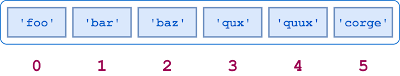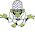### Python Data Structures - Lists in Python

In this series we will learn about non-primitive data structures in python.
We will not go over the common primitive data structures like int, booleans, floats and string but take a deeper look into non primitive data structures that help us in organizing data and appreciate python's powerful tools a little more. In this series we will go over 4 data-structures, those are
1. LIST
2. DICTIONARY
3. SET
4. TUPLELists in Python

We will learn through examples how to use them, what default methods python provides for them and which data structure to use according to our needs.

Let's go over Lists first:
Note: In case you are a video person, you can watch our full python tutorial here:

LIST

In python, Lists are similar to arrays in other languages. Lists don't always have to be homogeneous, and this it a very powerful tool for data storage in python. A single list can contain different Data types like Strings, Integers and even non primitive objects. Lists are also extremely useful for the implementation of stacks and queues. Unlike some other data structures in python that we will cover later, lists are mutable, and hence, they can be modified even after their creation.
Simply Speaking, lists are a type of container in Data Structures, which is used to store multiple data at a time. In python, lists are ordered and have a fixed count. The indexing of elements in a list are according to a definite sequence and the indexing start with 0 being the first element. Each element in the list has a fixed place in the list, this allows us to create duplicates of elements in the list, with each element having its own unique place.
Tip - Lists in python are a useful tool for preserving a sequence of data, later iterating over it and taking necessary steps. Lists can contain mutable elements unlike sets.

CREATING LISTS

Creation of Lists in Python is done by placing the sequence of data inside the square brackets[] separated by commas. Unlike Sets, we don't need a built-in function for creating list. A list can contain duplicate values with their different indexes and hence, multiple distinct or different values can be passed as a sequence we creating the list.
Let's look at the example below to understand the code better:

``` # Python program to demonstrate lists```
``` # Initializing a List List = [] print("Intialized a blank List: ") print(List) # Creating a List with the use of a String List = ['python lists'] print("\nList with String: ") print(List) # Creating a List with multiple values List = ["python", "list", "code"] print("\nList with multiple values: ") print(List) print(List) # Creating a Multi-Dimensional List (list inside a List) List = [['pytho', 'list'] , ['another list']] print("\nNested List: ") print(List) # Creating a List with # the use of Numbers # (Having duplicate values) List = [1, 2, 4, 4, 3, 3, 3, 6, 5] print("\nList with the use of Numbers: ") print(List) # Creating a List with multiple data types List = [5, 4, 'python', 3, 'list', 2, 1] print("\nList with the use of multiple datatypes: ") print(List) ```

To add elements in a list, we can use the built-in method append(). When using append() method, we can only add one element at the end of the list, to add multiple items using append(), we need to use loops. We can also add Tuples to the List with the using append method because tuples are immutable. Unlike Sets, We can also add lists to the existing list with the using the append() method.
One this to note about append() method is that it only works for the addition of elements at the end of the List, for addition of element at the any desired position, we can use the insert() method. Unlike append() which takes only one argument, we need to pass two parameters to the insert() method those are(position, value). Other than append() and insert() methods, there’s one more method for Addition of elements, extend(), this method can be used to add multiple elements at once at the end of the list.

Tip – append() & extend() methods can only add elements at the end, to add elements at desired position use the extend() method.

``` # Python program to demonstrate element addition in List```
``` # Creating a List List = [] print("Intialized blank List: ") print(List) # Addition of Elements # in the List List.append(5) List.append(4) List.append(3) print("\nList after Adding three elements: ") print(List) # Adding elements to the List using iterator for i in range(1, 4):    List.append(i) print("\nList after Addition of elements from 1-3: ") print(List) # Adding Tuples to the List List.append((5, 6)) print("\nList after adding a Tuple: ") print(List) # Adding list to a List List2 = ['Python', 'List'] List.append(List2) print("\nList after adding a List: ") print(List) # Adding Element at a specific Position # 12 in 4th position, python in first position List.insert(3, 12) List2.insert(0, 'Python') print("\nPerforming Insert Operation: ") print(List) # Addition of multiple elements at the end of list List.extend([8, 'Python', 'List]) print("\nList after Extend Operation: ") ```
``` print(List) ```

ACCESSING THE ELEMENTS OF A LIST

We can access the elements of a list using the index of the element in the list. The index operator [ ] is used to access an item in a list. The index of the list must be an integer. Elements in Nested lists are accessed using nested indexing.

``` # Creating a List with multiple values```
``` List = ["Python", "List"] # accessing an element from the list using index print("Accessing a element from the list") print(List)   print(List) # Creating a Multi-Dimensional List List = [['Python', 'List'] , ['List']] # accessing a element from the Multi-Dimensional List using print("Acessing a element from a Multi-Dimensional list") print(List) print(List) List = [1, 2, 'Python', 4, 'List', 6, 'Data'] # Accessing a element using negative indexing print("Element using negative indexing") # print the last element of list print(List[-1]) # print third from the last   print(List[-3]) ```
REMOVING ELEMENTS FROM THE LIST

In python lists Elements are removed from the List by using built-in remove() function. If the element doesn't exist then we get an Error message. Remove() method only removes one element at a time, we can use iterator to remove range of elements. Pop() function is also used to remove & return an element from the list, but by default it removes only the last element of the set, to remove element from a specific position in the List, we need to pass index of the element as an argument to the pop() method.

Tip – Remove method only removes the first occurrence of the searched element.

``` # Python program to demonstrate item removal from list```
``` # Creating a List List = [1, 2, 3, 4, 5, 6,          7, 8, 9, 10, 11, 12] print("Intialized a List: ") print(List) # Removing elements from List List.remove(5) List.remove(6) print("\nList after Removing two elements: ") print(List) # Removing elements from List using an iterator for i in range(1, 5):     List.remove(i) print("\nList after removal of a range of elements: ") print(List) # Removing element from the list using the pop() method List.pop() print("\nList after popping an element: ") print(List) # Removing element at a location from the list using the pop() method List.pop(3) print("\nList after popping an element at a specific: ") print(List) ```
SLICING A LIST
There are many ways to print a whole List with all elements in python, but for printing a specific range of elements from the list, we use need to use the Slice operation. We can perform the slice operation on Lists with the use of colon(:). To print elements from the start to a range use [:Index], to print elements from end use [:-Index], to print elements from specific Index till the end we use [Index:], to print elements within a range, we can use [Start Index:End Index] & to print whole List with the use of slicing operation, use [:]. Further, slicing can also be used to print whole List in reverse order like this: [::-1].

Tip – To print elements of List from the end, use Negative Indexes.

``` # Python program to demonstrate removing elements in a List```
``` # Creating a List List = ['G','E','E','K','S','F',        'O','R','G','E','E','K','S'] print("Intialized List: ") print(List) # Print elements in a specific range using Slice operation Sliced_List = List[2:7] print("\nSlicing elements in a range 2-7: ") print(Sliced_List) # Print elements from beginning to a predefined Sliced_List = List[:-5] print("\nElements sliced till 5th element from last: ") print(Sliced_List) # Print elements from a fixed point to end Sliced_List = List[5:] print("\nElements sliced from 5th "      "element till the end: ") print(Sliced_List) # Printing all elements Sliced_List = List[:] print("\nPrinting all elements of the list: ") print(Sliced_List) # Printing elements in reverse Sliced_List = List[::-1] print("\nPrinting List in reverse order: ") ```
print(Sliced_List)

In this tutorial we have seen how we can create list in python, add items to them, remove items from list, access list items and finally how to slice list items. This should get you pretty familiar with the Lists in Python. If you have any questions or comments, feel free to drop them down below. Enjoy python lists, happy coding.

1.